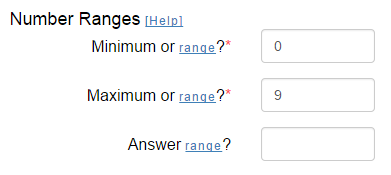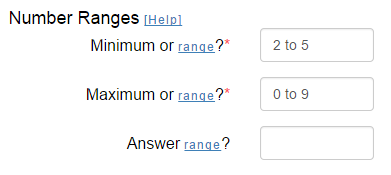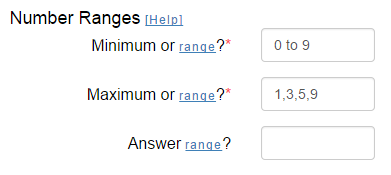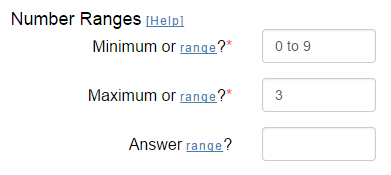# Basic Fact Help

#### Problem Type

There are 13 problem types:

2. Subtract
3. Multiply
4. Divide
6. Multiply / Divide
7. Add / Subtract / Multiply / Divide
8. Mixed
10. Subtract (Missing Number)
11. Multiply (Missing Number)
12. Divide (Missing Number)
13. Greater / Less Than

The first seven types are self-explanatory. The Mixed type allows a fact sheet to be created which contains a mixture of the basic arithmetic types. The Missing Number types will result in one of the problem numbers missing, but with the answer included. Lastly, a Greater / Less Than problem allows a student to determine the greater/less than relationship between two numbers.

#### Text Size

The text size that is used to create a fact sheet can be changed to allow more or less problems per page. By selecting a smaller size, more problems can normally be presented horizontally as well as vertically.

#### Options

The following are options for generating worksheets.
• Name/date/score - By enabling this option, each fact sheet will automatically have a page header which will include a space for name, date, and score. This header is not included on answer sheets.
• Number problems 1... - By selecting this option, all conversion problems will be numbered from 1 to the highest number.
• Don't allow answers of -1, 0, or 1 - If you would like to guarantee that no answer has a value of minus one, zero, or one, enable this option.
• Create numbers with two decimals - Although not frequently used, if you would like your problem facts to have up to two decimal places, enable this option.
• Extra solution space - By enabling this option, extra space will be included between each row for solving problems. The extra space is not included on answer sheets.
• No negative results for subtraction - By enabling this option, you are guaranteed that no subtraction problem will result in a negative answer (helpful for children who have not learned negative numbers yet). This option is only active when generating a Subtraction (and Missing Number) or Mixed problem type.
• Display problems horizontally - This option controls how the addition, subtraction, multiplication, and division problems are displayed. By selecting this option, problems will be presented horizontally (e.g. 3+5=8) rather than the default vertical layout where one number is beneath the other.
• Lock min/max positions - If you specify your minimum and maximum values as a range, this option controls whether those values remain locked as either the top or bottom (left or right) positions. If not, then the generator will randomly decide which position the numbers will appear in.

Note: Be aware that selecting this option can sometimes result in scenarios that cannot be resolved. For example, if you enable this option and select "No negative results for subtraction", a "Minimum or range" input value of 1 to 1, a "Maximum or range" input value of 5 to 10, you will receive an error (you cannot subtract a value 5-10 from 1 and get a positive result).

When selecting the problem type Division, you may select how to present the answers when creating an answer sheet. Selecting "Normal" will automatically show each answer up to five decimal places. Selecting "Whole" guarantees that each division problem will result in a whole number. The third choice, "Two-decimal rounding" will generate all answers with a rounded two-decimal answer. This option is only active when generating a Division (and Missing Number) or Mixed problem type.

#### Number Ranges

Creating a fact sheet problem requires the generation of random values (number1, number2). In order to do this, a numeric "range" is specified so that individual number values will be generated for each problem. There are three diffent ways to specify a range:

• Simple Range - Being the easiest to use to generate fact sheet problems, this method uses a single number range to select both number1 and number2. The low and high end of the range is entered as the "Minimum" and "Maximum" values. For example, if fact sheet problems should have number1 and number2 which are single-digits, the minimum and maximum values entered would be "0" and "9". Example:• Number Range - The number range method allows you to specify a sequential number range to be specified for number1 and/or number2. For example, if you would like fact sheet problems that always include a number from 2 to 5 as one number, and a single-digit number as the other number, the ranges would be "2 to 5" and "0 to 9" respectfully. Example:• List Range - This method allows you a largest amount of flexibility to tailor your fact sheet problems. For your input, specify a list of numbers to use in for number1 and/or number2; separate your list of numbers with commas. The generator will randomly select one of your list numbers. Example to practice a single digit number by single digit odd numbers:Another great example of using the List Range is to practice a specific fact family. To accomplish this, enter a Number Range along with your List Range (only include a singe number in your list...the fact to practice). Example to practice the 3 fact family: